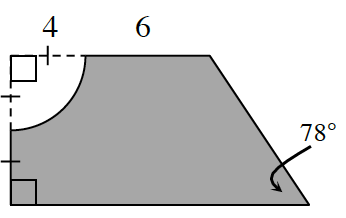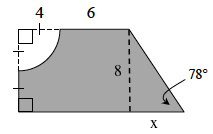### Home > CCG > Chapter 9 > Lesson 9.2.3 > Problem9-93

9-93.The Portland Zoo is building a new children’s petting zoo pen that will contain $6$ goats. One of the designs being considered is shown at right (the shaded portion). Assume the measurements are in meters.

1. What is the area of the petting zoo?

Find the area of the pen by first finding the area of the trapezoid. Notice the congruent segments in the right hand side. Then subtract the area of the quarter of the circle.

Draw a height to make a right triangle. The height is $8$ m. Use trigonometry to find the unknown side lengths.
Remember, the formula for the area of a circle is $A = r^2π$.$\text{tan}(78°)=\frac{8}{x}\\ x\cdot \text{tan}(78°)=8\\ x=\frac{8}{\text{ tan}(78°)}$

$\text{Area}=\left[\frac{1}{2}(\text{base}_{1}+\text{base}_{2})\text{height}\right]-[\text{radius}^{2}\pi\div 4]\\ \left[\frac{1}{2}((4+6)+\left ( 4+6+\frac{8}{\text{tan}(78°)} \right ))(4+4)\right]-[4^{2}\pi\div 4]\\ \frac{1}{2}\left(20+\frac{8}{\text{tan}(78°)}\right)8-(16\pi\div 4)\\ 80+\frac{32}{\text{tan}(78°)}-4\pi$

$≈ 74.24\text{ meters}^2$

2. How many meters of fence are needed to enclose the petting zoo area?

The formula for the circumference of a circle is $C = 2πr$. Remember it is only a quarter of a circle.

Meters of fencing needed is $≈ 36.2$ meters

3. What will be the density of goats in the pen? Show how you got your answer.